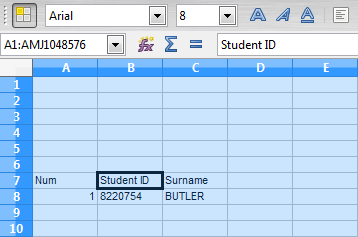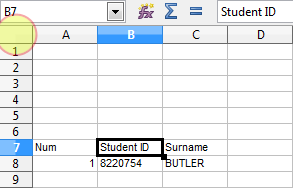# 07. Functions continued…

Functions really are where spreadsheets shine and so it’s worth us spending more time understanding how they work and putting some more complex ones into our current spreadsheet. This is what we’re going to get our spreadsheet to look like at the end of this section:While it might not look like much, we’ve actually got a bit to do in order to achieve this.

Cell B8
This one’s probably the easiest so let’s go ahead and fix it up. All you need is to have it refer to the appropriate cell over in the “gv” sheet, you can do that by following these steps:

1. Go to Cell B8
3. Click on the “gv” worksheet tab
4. Click on the first Student ID cell (should be D5)
5. Press “Enter

Cell A8
I’m going to introduce you to two new functions in this cell. First up is ROW. By putting the ROW function into a cell, it returns the number representing the row your cell is in. It may not seem important but the purpose of the “Num” column we’re inserting is to keep the numbers in rank order regardless of how the spreadsheet is sorted.

`=ROW()-7`

If we were to put that formula into Cell A8 it will give us the number 1. You can see we’ve subtracted 7 to ensure that our first student starts with the number 1 and not 8 as the ROW function would have returned. Notice also that the ROW function has no parameters and so the open and close brackets have nothing inside them.

The IF Function
This next function we’re going to encounter is probably one of the most important so I’ll spend some time ensuring it makes sense.

Consider what would happen if there were no students in the “gv” worksheet. Our formula in A8 would output the number 1 anyway. This spreadsheet would not be as versatile as it could be if it wasn’t able to automatically adapt to changes in “gv“. So what we’d like to do is something like this:

```IF there is student data THEN
output the number 1
OTHERWISE
don't output anything```

Spreadsheets can do calculations like this through the IF function. The only unanswered question though is how do we know if there is data in “gv“? This is what problem solving with computers is all about and so it’s worth trying to contemplate your own solution to that problem before reading on.

There are a number of ways we could do this step, one of the easiest is to just check the Student ID field…if it’s empty then there is no data. Now, in order to use the IF function we need to understand how it works:

`=IF(<<true/false question>>,<<true bit>>,<<false bit>>)`

The commas once again separate the parameters and so we now need to fit our ideas into a formula that will work. Try putting this into Cell A8:

`=IF(B8="","",ROW()-7)`

This now asks the question “Does Cell B8 have nothing in it“, if it does, then our cell is to contain nothing, if it doesn’t we use our ROW formula we worked out before.

We will end up using variants of this formula regularly throughout this tutorial, it’s a good way to have our spreadsheet only perform calculations that it needs to perform. If you want to further practice with it, try contemplating how you would use it to fix Cell C8 so that you only try extracting a surname when there is a name to work with!

Final Touches
Obviously you can fix up cells A7:B7 (notice how I refer to a range of cells, this will come up again so take note of it) and you may have noticed I’ve changed all the cells font size to 8. In order to do this, click on the light grey box that is located to the left of column A and above row 1:That box selects all the cells on your sheet…very handy! Then just change the font size to 8.

Okay, you may not think so, but I assure you, we’re properly getting somewhere now. Let’s get into a few more functions and formulae necessary to make this new sheet awesome!